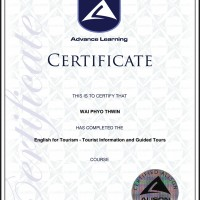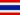# 4,2 Immissione dati

• Note di Apprendimento
• Revisione degli argomentiWAI P. 0 0 Formulas and functions. Formulas and functions are mathematical equations. The numeric value of formulas and functions automatically changes when the numeric value of variables associated with them changes.WAI P. 0 0 How many types of formula?
• Text Version